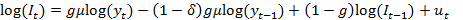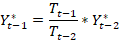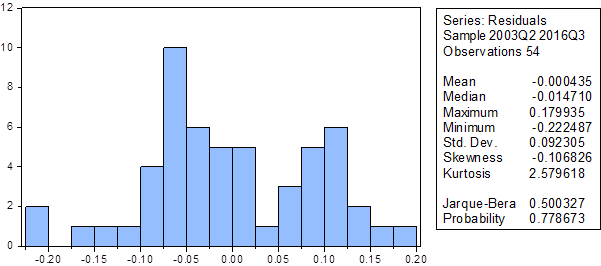Journal number 4 ∘ Giorgi Miqeladze
Investments accelerator and Tobins Model (Case of Georgia)

Summary

The article discusses the importance of the total capital in the process of total investment studies, calculation method of Georgia’s total capital and the opportunity of practical realization of above mentioned method. Research uses quarterly empirical data from 2003-2016 years.

The article empirically examines Modified case of L.M. Koyck’s investments accelerator model and Tobin’s investments model on example of Georgia. Practical models consider country’s economic specification and important events (results of the war in august, 2008 year).

Empirical studies validate interrelation of GDP’s and total investments’ current and lagged values with total capital. Also, research estimates quarterly values of Georgia’s total capital from 2003 year to 2016 year and depreciation rate, which is equal to 5.7%.

Key words: total investments; Georgia’s total capital; L. M.Koyck’s investments accelerator model; Tobin’s investments model; GDP.

Introduction

After the collapse of Soviet Union in the end of XX century 15 independent countries emerged. After gaining political independence, post-soviet countries faced various challenges. One of them was determination of economic policy. They had to choose strategy of transformation to market economy based on the countries’ non-existing statistical data. With the efforts of International Monetary Fund above mentioned process progressed according to the preliminary determined strategy in short period of time.

Nowadays, various statistical indicators exist for describing Georgia’s economy, however, still, there is no information about such important indicator as volume of total capital. As investments are determined by the total capital, lack of stated information hinders building total investments model on Georgia’s economy. Most of the investments models in economic theory use volume of total capital as a factor variable.

Investments Accelerator Model

There is no statistical information about volume of total capital in Georgia. Accordingly, investments accelerator model, which does not include volume of capital as a variable is L.M. Koyck’s model (Bernd Ernst R., 2005):(1)

where It  represents current value of investments, It-1 – lagged value of investments, Yt - current value of GDP, Yt-1 - lagged value of GDP, δ - depreciation rate of total capital, g - adjustment rate to desirable capital and, lastly, μ – ratio of desirable capital and GDP.

This model is based on several assumptions: 1) ratio of desirable capital and output is constant over time; 2) depreciation rate is not different time periods; 3) currents capital’s adjustment rate to desirable capital is constant.  For the estimation of investments accelerator model on case of Georgia it is optimal using modified variant of (1) model, where variables will be represented in logarithms. Particularly, we step from the additive connection among model variables to multiplicative connection. Stated assumption is based on connection between investments and growth rate of output in Georgian economy. Finally, model will take the following form:(2)

for the realization of expression (2) quarterly data of total investments and output is used from 2003 – 2016 years.

Nominal volume of investments is divided by GDP deflator for deriving investments real volume (GDP deflator is the ratio of nominal GDP and real GDP).

Time series include seasonal components. Based on the variables’ characteristics, for elimination of seasonal components the article uses multiplicative method of seasonal adjustment (Census X12) implemented by the bureau of population census of USA.

Time series should be tested whether they are TSP or DSP type by the procedure suggested by J. J. Dolado, T. Jenkinson and S. Sosvilla-Rivero. Particularly, TSP type implies that series are nonstationary because of variable trend, whereas DSP type implies nonstationarity because of variable variance (Dolado, Juan J., Tim Jenkinson, and Simon Sosvilla‐Rivero, 1990). Using Dickey-Fuller test for all four series null hypothesis is derived about having unit root, which implies their nonstationarity (Dickey, David A., and Wayne A. Fuller.,1979). Using differences for making series stationary identify that all four series are integrated of first order I(1).

Risk of false regression may arise while building model (2), because model’s series belong to nonstationary integrated of first order series.

Engle-Granger test checks the risk of false regression by testing the co integration between the values of total investments and output. Null hypothesis of Engle-Granger test implies absence of co integration between series, whereas according to alternative hypothesis co integration exists between series (Ananiashvili, I.2014).  Alternative hypothesis is approved with the 1% significance level about the existence of co integration between investments and output. While using above mentioned tests, lag length is 4, because quarterly data is used for the realization of the model.

Distribution of (2) model’s residual members estimated by using least square method will have the following form:

Table 1: Distribution of (2) model’s residual membersAs the histogram shows, residual members do not have normal distribution. However, by further observation it is possible to make the following conclusion: distribution of residual members is very much alike normal distribution by excluding one outlier value. For determining this fact in (2) model it is desirable to estimate model coefficients and determine, which gives the opportunity to calculate residual members for each quarter.

(2) model by estimating with least square method will have the following form:

Table 2: Regression Results of model (2)

 Dependent Variable: LOG_INVESTMENT_REAL_SA Method: Least Squares Date: 03/04/17   Time: 16:24 Sample (adjusted): 2003Q2 2016Q3 Included observations: 54 after adjustments Convergence achieved after 1 iteration LOG_INVESTMENT_REAL_SA=(1-C(1))*LOG_INVESTMENT_REAL_1_SA +C(1)*C(2)*LOG_GDP_REAL_SA-(1-C(3))*C(1)*C(2) *LOG_GDP_REAL_1_SA Coefficient Std. Error t-Statistic Prob. C(1) 0.233604 0.078983 2.957634 0.0047 C(2) 19.27548 7.683212 2.508778 0.0153 C(3) 0.042707 0.017561 2.431847 0.0186 R-squared 0.782626 Mean dependent var 7.232155 Adjusted R-squared 0.774101 S.D. dependent var 0.339574 S.E. of regression 0.161395 Akaike info criterion -0.755968 Sum squared resid 1.328470 Schwarz criterion -0.645469 Log likelihood 23.41113 Hannan-Quinn criter. -0.713353 Durbin-Watson stat 2.146285(3)

g= 0.233604, μ =19.27548,  δ = 0.042707

Residual members’empirical values have the following form:

Table 3: Residual members values of model (3)

 Year Quarter Year Quarter Year Quarter 2003 2 -0.08466 2007 4 -0.17926 2012 2 0.019044 2003 3 -0.08202 2008 1 -0.016 2012 3 -0.0414 2003 4 -0.04632 2008 2 0.079245 2012 4 0.054094 2004 1 0.222127 2008 3 0.023866 2013 1 -0.03716 2004 2 -0.04499 2008 4 0.104529 2013 2 -0.01781 2004 3 0.005715 2009 1 -0.91364 2013 3 -0.02089 2004 4 -0.02772 2009 2 -0.06345 2013 4 -0.08965 2005 1 -0.05906 2009 3 0.119321 2014 1 0.185331 2005 2 0.106004 2009 4 -0.05967 2014 2 0.10849 2005 3 -0.00439 2010 1 -0.14261 2014 3 -0.01204 2005 4 0.08056 2010 2 0.113044 2014 4 0.143231 2006 1 -0.03323 2010 3 0.058623 2015 1 0.140492 2006 2 -0.05169 2010 4 -0.01266 2015 2 -0.01819 2006 3 -0.16025 2011 1 -0.05357 2015 3 0.067159 2006 4 0.078995 2011 2 -0.04132 2015 4 0.155113 2007 1 0.089376 2011 3 0.126777 2016 1 0.105341 2007 2 -0.21704 2011 4 -0.04932 2016 2 0.053521 2007 3 0.145506 2012 1 0.119419 2016 3 0.041622

Outlier value of residual members occurs in the first quarter of 2009 year. Economic interpretation of this fact can be easily found in the nearest history of Georgia. Particularly, Georgia had a war in third quarter of 2008 year, which had short-term economic effects in the first quarter of 2009 year.

Remark: August war had a short term economic effect on Georgia, because grants from world organizations and partner countries nullified long term economic effect.

It is desirable to include factor variable in (2) model which will characterize effects of the war. This will be binary variable with the values of 0 in all the time periods except the first quarter of 2009 year, where the value is 1. After taking into consideration stated modifications and empirical estimation, the model will take the following form:

Table 4: Regression results of model (4)

 Dependent Variable: LOG_INVESTMENT_REAL_SA Method: Least Squares Date: 07/30/17   Time: 14:11 Sample (adjusted): 2003Q2 2016Q3 Included observations: 54 after adjustments Convergence achieved after 11 iterations LOG_INVESTMENT_REAL_SA=(1-C(1))*LOG_INVESTMENT_REAL_1_SA +C(1)*C(2)*LOG_GDP_REAL_SA-(1-C(3))*C(1)*C(2) *LOG_GDP_REAL_1_SA+C(4)*WAR Coefficient Std. Error t-Statistic Prob. C(1) 0.236232 0.046509 5.079285 0.0000 C(2) 14.64838 3.788997 3.866031 0.0003 C(3) 0.057283 0.015086 3.797066 0.0004 C(4) -0.959774 0.097405 -9.853411 0.0000 R-squared 0.926108 Mean dependent var 7.232155 Adjusted R-squared 0.921675 S.D. dependent var 0.339574 S.E. of regression 0.095035 Akaike info criterion -1.797950 Sum squared resid 0.451585 Schwarz criterion -1.650618 Log likelihood 52.54466 Hannan-Quinn criter. -1.741130 Durbin-Watson stat 2.520187(4)

g= 0.236232, μ =11.64838,  δ = 0.057283

The distribution of the residual members in model (4) is normal.

Table 5: Distribution of (4) model’s  Residual membersDurbin-Watson test is not recommended for checking autocorrelation in residual member, because model assumptions are violated: model does not contain a constant member and, also, model includes lagged values of dependent variable as a factor variable. Breusch-Godfrey’s Lagrange multiplier (LM) test is recommended for checking serial correlation in residual member. According to the null hypothesis of LM test there is no autocorrelation in residual member, whereas alternative hypothesis states that there is serial correlation in residual member (Breusch, T. S.,1978; Godfrey, L. G.,1978) . Breusch-Godfrey test results show that null hypothesis is statistically significant, which states that there is no serial correlation in residual member.

While checking heteroscedasticity in residual member with Glejser null hypothesis is accepted with 99% confidence interval, which means existence of homoscedatsticity (Glejser, Herbert.,1969). Accordingly, variance of residual member is constant.

It is shown that all the coefficients of model (4) are statistically significant, as for all of them H1 hypothesis of T-test is accepted for statistical significance (Ananiashvili, I.,2010). Also, coefficients of determination and corrected determination have quite high values - 0.93 and 0.92 accordingly, which shows that model variation is well explained by model variables. Also, F=153.53, Fcr=3.73, F> Fcr shows that model is valid.

If we take into account  and capital consumption values, which is , calculation of capital volume in different time periods is possible.

Table 6: Total real capital values

 Year Quarter Total capital consumption GDP deflator Total real capital consumption Total real capital 2003 I 192.77 0.65 295.08 5151.35 2003 II 226.98 0.63 360.03 6285.15 2003 III 247.42 0.61 404.98 7069.86 2003 IV 265.76 0.59 449.73 7851.01 2004 I 221.35 0.67 330.27 5765.51 2004 II 238.75 0.68 349.88 6107.87 2004 III 248.94 0.67 370.04 6459.82 2004 IV 267.78 0.66 406.85 7102.46 2005 I 235.28 0.77 307.02 5359.65 2005 II 270.29 0.72 375.38 6553.03 2005 III 296.18 0.70 420.81 7346.11 2005 IV 311.71 0.71 436.26 7615.95 2006 I 271.36 0.79 342.17 5973.36 2006 II 309.73 0.80 387.35 6762.05 2006 III 348.10 0.77 454.89 7941.05 2006 IV 377.18 0.78 480.74 8392.38 2007 I 334.31 0.86 390.32 6813.89 2007 II 392.90 0.86 457.95 7994.52 2007 III 426.20 0.84 507.55 8860.40 2007 IV 463.61 0.88 526.91 9198.38 2008 I 381.77 0.95 402.46 7025.85 2008 II 440.39 0.96 459.60 8023.37 2008 III 475.87 0.93 509.94 8902.03 2008 IV 460.02 0.93 495.94 8657.80 2009 I 379.57 0.94 402.10 7019.55 2009 II 422.11 0.91 465.20 8121.15 2009 III 452.30 0.92 494.29 8628.90 2009 IV 497.39 0.92 539.79 9423.21 2010 I 460.93 1.00 460.93 8046.59 2010 II 512.60 1.00 512.60 8948.51 2010 III 549.25 1.00 549.25 9588.36 2010 IV 604.01 1.00 604.01 10544.25 2011 I 619.37 1.13 548.39 9573.33 2011 II 712.17 1.09 650.41 11354.37 2011 III 636.89 1.08 589.56 10292.09 2011 IV 692.33 1.08 639.68 11167.00 2012 I 687.83 1.16 593.02 10352.40 2012 II 774.41 1.11 694.81 12129.39 2012 III 653.87 1.09 601.88 10507.10 2012 IV 680.87 1.08 631.35 11021.54 2013 I 703.83 1.15 609.75 10644.48 2013 II 767.92 1.11 693.30 12103.14 2013 III 656.30 1.07 610.84 10663.51 2013 IV 681.46 1.07 636.20 11106.32 2014 I 717.29 1.17 611.30 10671.65 2014 II 843.22 1.16 728.78 12722.45 2014 III 712.08 1.13 632.61 11043.59 2014 IV 739.38 1.11 664.96 11608.29 2015 I 748.32 1.24 602.78 10522.85 2015 II 920.41 1.23 748.13 13060.26 2015 III 789.01 1.20 658.26 11491.29 2015 IV 815.78 1.17 698.70 12197.25 2016 I 802.47 1.29 620.59 10833.82 2016 II 987.32 1.24 799.01 13948.38 2016 III 864.01 1.24 698.16 12187.87

source: National statistic office of Georgia, total capital consumption.

At first, Total real capital consumption is calculated considering GDP deflator. Next step evaluates total capital real values, which is the ratio of total capital real consumption and depreciation rate.

Estimate results of model (4) are statistically valid, because there is no autocorrelation in residual member, which shows that model does not omit statistically significant independent variables and model has right functional specification. Residual member has constant variance, coefficients and the model are statistically significant. Moreover, there is low correlation level among the variables.

According to model (4) investments’ current value is positively related to the lagged value of investments. Growth in lagged value of investments stimulates investments in next period, particularly 1% growth in lagged value of investment increases investments of current period by 0.76%. Also, logically, output growth in current period increases current investment values. Output growth by 1% in current period increases investments by 3.46%. Current period’s investment volume is negatively related with output value of previous period.1% increase of the lagged value of output decreases investments values of current period by 3.26%. This negative relationship takes into consideration delayed process of investments’ realization. Investments that are planned in previous time period and are realized in current time period decrease investments in current period, because companies try to prevent increase of investments cost in short term. The war factor has a negative value, which means that the war is negatively related to total investments’ volume. Logically, period during the war the volume of total investments decreases in short term.

Tobin’s investments model Based on the USA’s economy R. Kopke empirically tested Tobin’s investments model, which had the following form (Bernd Ernst R.,2005):(5)

where It is the volume of total investments, Kt – total capital values, qt - Tobin’s coefficient in respective time period and bj – coefficient.

It should be mentioned that empirical realization of Tobin’s investments model on Georgian economy case faces following problems:  1) there is no information about Tobin’s coefficient – q; 2) there is no empirical data on total capital. The later can be fixed by using the result from investments accelerator model, which estimates total capital values. However, because of the absence of Tobin’s coefficient values statistically valid model cannot be built on Georgian case.

Remark: Calculation of Tobin’s coefficient or replacing it with economically similar factor is not possible, because there are no economic indicators that could be used for fixing stated problem. Also, considering that technical estimation problem in model (5) should be fixed by assuming that Tobin’s coefficient is constant through time and creating new coefficients  has no valid economic logic, because above mentioned fact confronts Tobin’s theory.

Conclusion

Investments accelerator model well reflects Georgian economy’s reality. According to this model, investments are positively related to the lagged values of investments and current value of GDP. This result is in accordance with the economic theory. Also, increase in lagged value of GDP decreases investments values in current period. This relationship reflects delayed process of investments’ realization. Investments that are planned in previous time period and are realized in current time period decrease investments in current period, because companies try to prevent increase of investments cost in short term.

Depreciation rate of Georgia’s total capital is 5.7% and volume of total capital in last period is more than 12 billion Georgian Lari, which is approximately 5 billion US dollars.

The war in August, 2008 year had a short term negative effects on Georgia’s total investments, which is proved by the investments accelerator model.

During the realization of Tobin’s investments model on example of Georgian economy it is possible to use estimated values of total capital from investments accelerator model and to assume that using twice regressive model will not increase statistical errors. However, it is not recommended to use Tobin’s investments model on example of Georgian economy, because there is no empirical data about Tobin’s coefficient. Moreover, there is no economic variable that could be used as a replacement in the model. It is not economically reasonable to use the assumption that Tobin’s coefficient is constant through time for fixing above mentioned problem, because it confronts Tobin’s theory.

References

1. Ananiashvili, I. (2014).Timeline series analysis. TSU, Tbilisi.
2. Ananiashvili, I. (2010). Econometrics.Meridiani,Tbilisi.
3. Bernd Ernst R. (2005). “Classical and modern”. Applied  econometric. Moscow, Iuniti-dana,847.
4. Breusch, Trevor S. (1978). "Testing for autocorrelation in dynamic linear models." Australian Economic Papers 17.31, 334-355.
5. Dickey, David A., and Wayne A. Fuller. (1979). "Distribution of the estimators for autoregressive time series with a unit root." Journal of the American statistical association 74.366a, 427-431.
6. Dolado, Juan J., Tim Jenkinson, and Simon Sosvilla‐Rivero. (1990). "Cointegration and unit roots." Journal of economic surveys 4.3, 249-273.
7. Glejser, Herbert. (1969). "A new test for heteroskedasticity." Journal of the American Statistical Association 64.325, 316-323.
8. Godfrey, Leslie G. (1978). "Testing against general autoregressive and moving average error models when the regressors include lagged dependent variables." Econometrica: Journal of the Econometric Society,  1293-1301.
9. National statistic office of Georgia, Total capital consumption, available from: http://geostat.ge/index.php?action=page&p_id=119&lang=eng (Accessed 29 July 2017).